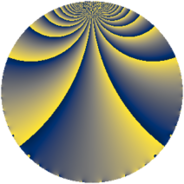# Properties

 Label 429.2.mLevel $429$ Weight $2$ Character orbit 429.m Rep. character $\chi_{429}(109,\cdot)$ Character field $\Q(\zeta_{4})$ Dimension $56$ Newform subspaces $2$ Sturm bound $112$ Trace bound $3$

# Related objects

## Defining parameters

 Level: $$N$$ $$=$$ $$429 = 3 \cdot 11 \cdot 13$$ Weight: $$k$$ $$=$$ $$2$$ Character orbit: $$[\chi]$$ $$=$$ 429.m (of order $$4$$ and degree $$2$$) Character conductor: $$\operatorname{cond}(\chi)$$ $$=$$ $$143$$ Character field: $$\Q(i)$$ Newform subspaces: $$2$$ Sturm bound: $$112$$ Trace bound: $$3$$ Distinguishing $$T_p$$: $$2$$

## Dimensions

The following table gives the dimensions of various subspaces of $$M_{2}(429, [\chi])$$.

Total New Old
Modular forms 120 56 64
Cusp forms 104 56 48
Eisenstein series 16 0 16

## Trace form

 $$56q + 56q^{9} + O(q^{10})$$ $$56q + 56q^{9} + 4q^{11} + 48q^{14} - 8q^{15} - 72q^{16} - 24q^{20} - 40q^{22} + 8q^{26} + 32q^{31} - 4q^{33} - 16q^{34} - 24q^{37} - 48q^{42} + 12q^{44} - 48q^{47} + 32q^{48} + 32q^{55} - 48q^{58} - 40q^{59} - 8q^{60} + 24q^{66} + 8q^{67} - 40q^{70} - 48q^{71} + 16q^{78} + 48q^{80} + 56q^{81} + 40q^{86} - 72q^{89} - 72q^{91} + 64q^{92} - 16q^{93} + 4q^{99} + O(q^{100})$$

## Decomposition of $$S_{2}^{\mathrm{new}}(429, [\chi])$$ into newform subspaces

Label Dim. $$A$$ Field CM Traces $q$-expansion
$$a_2$$ $$a_3$$ $$a_5$$ $$a_7$$
429.2.m.a $$28$$ $$3.426$$ None $$0$$ $$-28$$ $$4$$ $$0$$
429.2.m.b $$28$$ $$3.426$$ None $$0$$ $$28$$ $$-4$$ $$0$$

## Decomposition of $$S_{2}^{\mathrm{old}}(429, [\chi])$$ into lower level spaces

$$S_{2}^{\mathrm{old}}(429, [\chi]) \cong$$ $$S_{2}^{\mathrm{new}}(143, [\chi])$$$$^{\oplus 2}$$